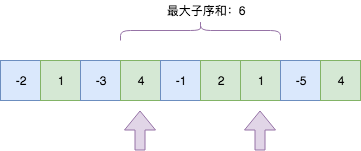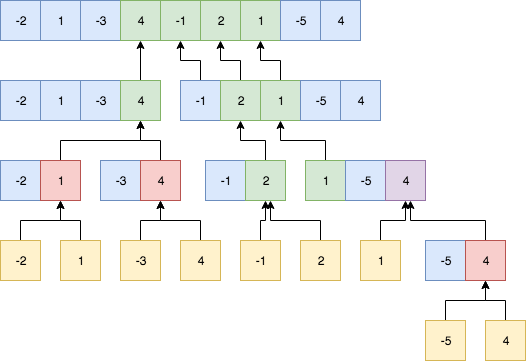# 最大子序和 Maximum Subarray

## 题目

Given an integer array nums, find the contiguous subarray (containing at least one number) which has the largest sum and return its sum.

Example:

``````Input: [-2,1,-3,4,-1,2,1,-5,4],
Output: 6
Explanation: [4,-1,2,1] has the largest sum = 6.
``````

If you have figured out the O(n) solution, try coding another solution using the divide and conquer approach, which is more subtle.

## 思路&题解

1. 我们顺序从第一个元素开始计算最大子集和；
2. 然后将每一轮取较大的一个；
3. 最终得到整个数组的最大连续和。
``````public int maxSubArray(int[] nums) {
int max = nums;
for (int i = 0; i < nums.length; i++) {
int sum = nums[i];
int sumMax = sum;
for (int j = i + 1; j < nums.length; j++) {
sum += nums[j];
sumMax = Math.max(sumMax, sum);
}
max = Math.max(max, sumMax);
}
return max;
}
``````

[!NOTE] 执行用时 : 148 ms, 在Maximum Subarray的Java提交中击败了5.08% 的用户

O(n)优化版本

1. 连续的子集要保持和最大，肯定尽量保持都是正数，正数有有利于和增大；
2. 如果存在负数，则必须是负数后面有正数，可以抵消，负数的负面作用；
3. 负数不可能是连续子集的开头或者结尾，最多存在于中间位置；

1. 当前和如果大于0，可以继续累加；如果小于0，则抛弃，从下一个位置继续求解；
2. 在循环中，同时记录当前求和的值和最大和，用于保证`特征2: 如果存在负数，则必须是负数后面有正数，可以抵消，负数的负面作用```````public int maxSubArray(int[] nums) {
int max = nums;
int sum = 0;
for (int i = 0; i < nums.length; i++) {
if (sum > 0) {
sum += nums[i];
} else {
sum = nums[i];
}
max = Math.max(max, sum);
}
return max;
}
``````

[!NOTE] 执行用时 : 3 ms, 在Maximum Subarray的Java提交中击败了78.21% 的用户

O(nlgn)分治法

1. 连续的子序列正好全部在前半截数组；
2. 连续的子序列正好全部在后半截数组；
3. 连续的子序列在左右数组内个存在一部分，完整序列为左右两部分加和；``````public int maxSubArray(int[] nums) {
return maxSub(nums, 0, nums.length - 1);
}

public int maxSub(int[] nums, int left, int right) {
if (left == right) {
return nums[left];
}
int mid = (left + right) / 2;
int leftSum = maxSub(nums, left, mid);
int rightSum = maxSub(nums, mid + 1, right);

int leftSumPart = 0;
int tmpSum = 0;
for (int i = mid; i >= left; i--) {
tmpSum += nums[i];
if (tmpSum >= leftSumPart || leftSumPart == 0) {
leftSumPart = tmpSum;
}
}

int rightSumPart = 0;
tmpSum = 0;
for (int i = mid + 1; i <= right; i++) {
tmpSum += nums[i];
if (tmpSum >= rightSumPart || rightSumPart == 0) {
rightSumPart = tmpSum;
}
}
return Math.max(Math.max(leftSum, rightSum), leftSumPart + rightSumPart);
}
``````

[!NOTE] 执行用时 : 5 ms, 在Maximum Subarray的Java提交中击败了66.78% 的用户